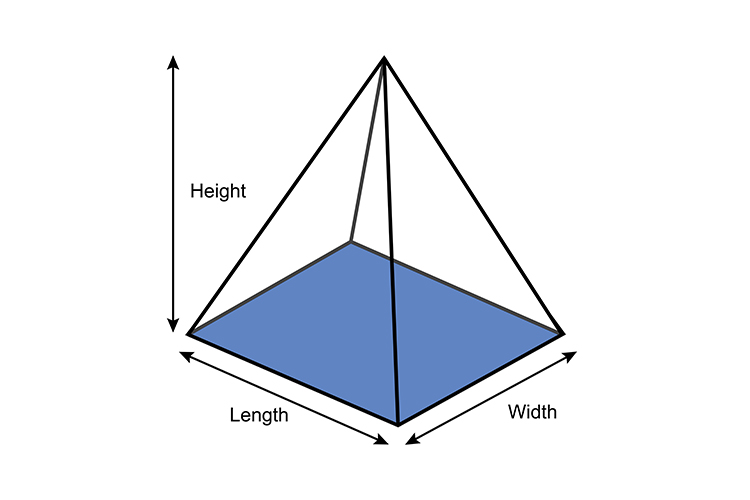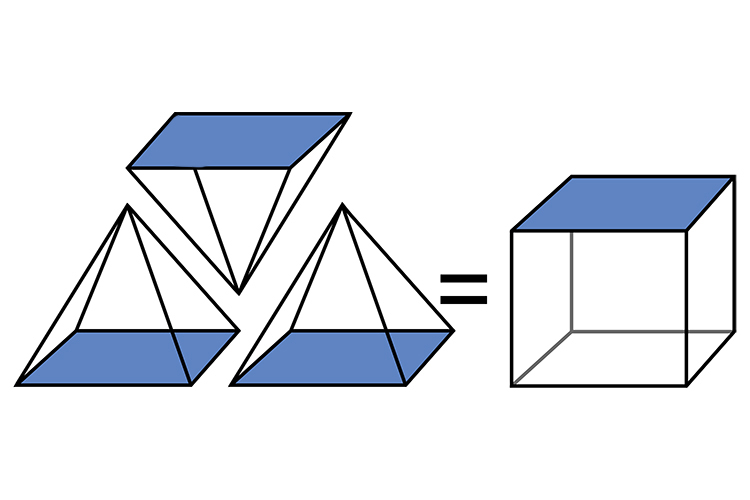# Volume of square based pyramids

The volume of a square based pyramid works on area of the base multiplied by height and some factor.Base area of square based pyramid = Len\g\th\times\width

Volume of a square based pyramid = 1/3times\area\ of\ base\ timesheight

Volume of a square based pyramid = 1/3times\l\e\n\g\th\times\width\timesheight3 square based pyramids fill 1 cube (with the same size base and height).

NOTE:

Areas shaded blue are all equal.